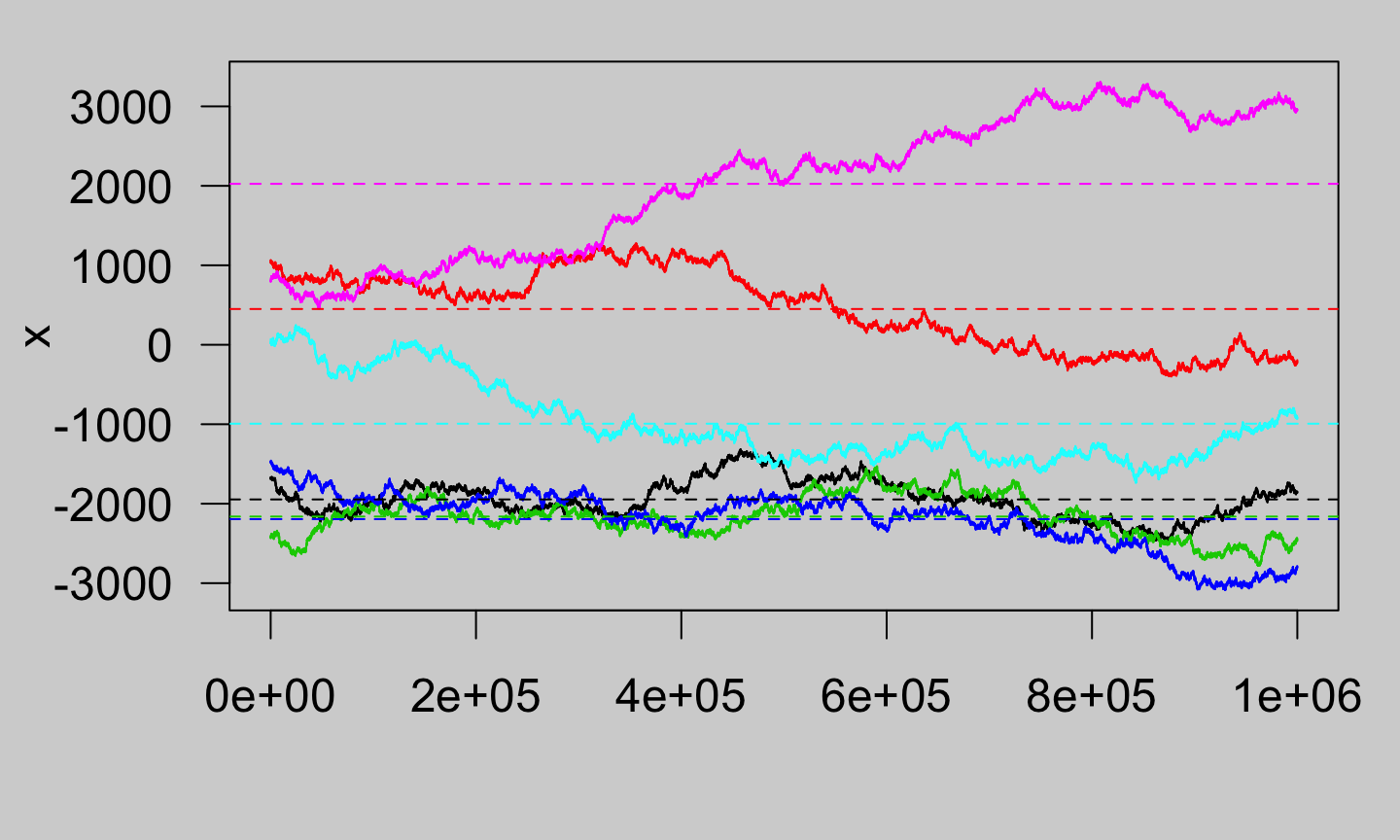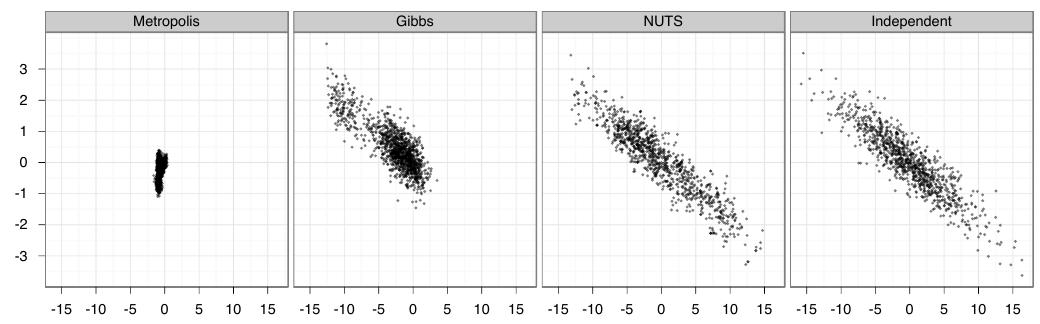June 27, 2016

## Installation

• If you have not installed the rstan R package yet, please follow the steps at https://github.com/stan-dev/rstan/wiki/RStan-Getting-Started because you need Xcode on a Mac, RTools on Windows, or build-essential on Linux
• If you have installed the rstan R package, please make sure packageVersion("rstan") is 2.10.1.
• In either case, please verify that a Stan program compiles and runs with
example("stan_model", package = "rstan", run.dontrun = TRUE)
• Also, verify that packageVersion("rstanarm") is 2.10.1; otherwise execute
install.packages("rstanarm", repos = "https://cloud.r-project.org", dependencies = TRUE)
* If you have Windows and R version less than 3.3.x, you may have to add type = "source"

## Outline

1. Probability background, Markov Chain Monte Carlo, rstanarm example
2. Break from 2:15 to 2:30
3. Hierarchical model example, Stan language, writing Stan programs

## Obligatory Disclosure

• I am an employee of Columbia University, which has received several research grants to develop Stan
• I am also a cofounder of Stan Group (http://stan.fit), which provides support, consulting, etc. for businesses using Stan
• According to Columbia University policy, any such employee who has any equity stake in, a title (such as officer or director) with, or is expected to earn at least $$\5,000.00$$ per year from a private company is required to disclose these facts in presentations

## What Was the Probability of Brexit?

• If I were to have asked you (a week ago), what is the probability that the British would vote to leave the European Union, what would you have said?
• If everyone could have supplied an answer to that question (a week ago), where did these beliefs about the probability of this event come from?

## Different Perspectives on Probability

What is the paradigm? What is fixed? What is random? What proportion is important? What is the conclusion?
Randomization $${y_1, y_2, \dots, y_N}$$ Treatment assignment $$p$$-value for null: ATE $$= 0$$? ATE $$\neq 0$$
Frequentist $$Y$$, $$\boldsymbol{\theta}$$, $$N$$ Sample inclusion $$\theta \in$$ confidence intervals (plural) Something basically Bayesian
Supervised learning $${y_1, y_2, \dots, y_N}$$ Training / testing inclusion Correctly classified outcomes in testing data Some procedure predicts best
Bayesian $${y_1, y_2, \dots, y_N}$$, $$\boldsymbol{\theta}$$ Beliefs about $$\boldsymbol{\theta}$$ Posterior draws of $$\theta \in \left(a,b\right)$$ Decision or action

## Two Justifications for Bayes Rule

1. $$f\left(\mathbf{y}\right) \times f\left(\boldsymbol{\theta} | \mathbf{y}\right) = f\left(\boldsymbol{\theta}, \mathbf{y}\right) = f\left(\boldsymbol{\theta}\right) \times f\left(\mathbf{y} | \boldsymbol{\theta}\right) \implies f\left(\boldsymbol{\theta} | \mathbf{y}\right) = \frac{f\left(\boldsymbol{\theta}\right) \times f\left(\mathbf{y} | \boldsymbol{\theta}\right)}{f\left(\mathbf{y}\right)}$$ where $$\mathbf{y} = \{y_1, y_2 \dots y_N\}$$ and $$f\left(\cdot\right)$$ is a PDF so $$f\left(\cdot\right) \geq 0$$ & $$\int f\left(u\right)du = 1$$
• $$f\left(\boldsymbol{\theta}\right)$$ represents what someone believes about $$\boldsymbol{\theta}$$ prior to observing $$\mathbf{y}$$
• $$f\left(\boldsymbol{\theta} | \mathbf{y}\right)$$ represents what someone believes about $$\boldsymbol{\theta}$$ after observing $$\mathbf{y}$$
• $$f\left(\mathbf{y} | \boldsymbol{\theta}\right)$$ is the likelihood function, a function of $$\boldsymbol{\theta}$$ for an observed $$\mathbf{y}$$
• $$f\left(\mathbf{y}\right) = \int \cdots \int \int f\left(\boldsymbol{\theta}\right) f\left(\mathbf{y} | \boldsymbol{\theta}\right) d\theta_1 d\theta_2 \dots d\theta_K = \mathbb{E}_{\boldsymbol{\theta}}f\left(\mathbf{y} | \boldsymbol{\theta}\right)$$
2. $$f\left(\boldsymbol{\theta} | \mathbf{y}\right)$$ is the unique function that minimizes the sum of
• Penalty: Kullback-Leibler divergence to $$f\left(\boldsymbol{\theta}\right)$$
• Expected misfit: $$\mathbb{E}_{\boldsymbol{\theta}}\left[-\ln f\left(\mathbf{y} | \boldsymbol{\theta}\right)\right]$$

## Markov Chain Monte Carlo

• Even if $$f\left(\mathbf{y}\right)$$ could be calculated, you would have to do another K-dimensional integral to obtain something like $$\mathbb{E}\left[\theta_k | \mathbf{y}\right]$$
• So we draw randomly $$S$$ times from the posterior distribution — which does not require knowing $$f\left(\mathbf{y}\right)$$ — and estimate $$\mathbb{E}\left[\theta_k | \mathbf{y}\right]$$ with $$\frac{1}{S}\sum_{s=1}^S{\tilde{\theta}_k^{[s]}}$$
• There is no way to draw independently from most posterior distributions
• The price to be paid for relying on Markov Chain Monte Carlo (MCMC) to draw from a posterior distribution is that the draws are not independent
• The degree of dependence in a MCMC algorithm governs how badly $$\frac{1}{S}\sum_{s=1}^S{g\left(\widetilde{\boldsymbol{\theta}}^{[s]}\right)}$$ estimates $$\mathbb{E}g\left(\boldsymbol{\theta}\right) | \mathbf{y}$$ for finite $$S$$
• Effective Sample Size is a concept like that in complex survey design and is defined as the number of independent draws that would estimate a posterior mean with the same precision as the $$S$$ dependent draws you do have

## A Markov Process with Severe Dependence

par(mar = c(4,4,1,1) + .1, las = 1, bg = "lightgrey")
x <- sapply(1:6, FUN = function(i) arima.sim(model = list(ar = 0.9999999), n = 10^6))
matplot(x, type = "l", col = 1:6, lty = 1)
for (j in 1:ncol(x)) abline(h = mean(x[,j]), col = j, lty = 2)## Why Doesn't Everyone Use Bayesian Methods?

• There are very few useful analytical results
• Can't let 1 programmer write generic code that all paying researchers use
• Posterior distribution depends not just on the researcher's data but on the prior beliefs of the researcher, which must be encoded somehow
• To express your prior beliefs using probability distributions, you need to know the functional characteristics of lots of probability distributions
• Drawing from an entire probability distribution is a much more ambitious task than finding a optimal point and takes a lot longer
• Many researchers were frustrated by the BUGS family of software
• Harder to publish a Bayesian analysis in an applied journal

## What is Stan and How Does It Help?

• Includes a probabalistic programming language
• The rstanarm, brms, and rethinking R packages provide code to specify some statistical models — with a limited choice of prior distributions — that can be mapped into the Stan language
• Includes new Hamiltonian Monte Carlo (HMC) algorithms
• HMC is to MCMC as BFGS is to optimization
• HMC is aided by the gradient of the posterior distribution wrt $$\boldsymbol{\theta}$$
• Dependence between consecutive draws is minimal
• Includes a matrix and scalar math library that supports autodifferentiation
• Includes interfaces from R and other high-level software
• Includes (not Stan specific) post-estimation R functions of MCMC output
• Includes a large community of users and many developers

## Overview of Hamiltonian Monte Carlo

• Since the early 1990s, most MCMC uses Gibbs updates when feasible and falls back to something more general otherwise
• Gibbs entails drawing $$\theta_k$$ from its "full-conditional distribution": $$\theta_k | \boldsymbol{\theta}_{-k}, \mathbf{y}$$
• "Something more general" includes slice sampling, Metropolis-Hastings, etc., which is needed when the full-conditional distribution of $$\theta_k$$ is not known in closed form
• If Gibbs updates are feasible, they are easy to code and fast to execute but are statistically inefficient because the dependence between draws is high
• HMC differs from Gibbs in that all elements of $$\boldsymbol{\theta}$$ are updated simultaneously
• H stands for Hamiltonian, which is a physics framework for how a particle $$\left(\boldsymbol{\theta}\right)$$ moves through an unbounded frictionless space

## Example of Drawing from a Multivariate Normal

• $$\mathbf{y} \thicksim \mathcal{N}_{250}\left(\mathbf{0}, \boldsymbol{\Sigma}\right)$$ where $$\boldsymbol{\Sigma}$$ is ill-conditioned but focus on just two dimensions
• Do 1 million draws w/ Random Walk Metropolis & Gibbs, thinning by $$1000$$
• Do 1000 draws with the NUTS algorithm in Stan and 1000 independent drawsComparison of MCMC Samplers

## Details of Hamiltonian Monte Carlo

• HMC augments the parameter space with a momentum vector $$\left(\boldsymbol{\phi}\right)$$ of size $$K$$
• $$\boldsymbol{\phi}$$ does not enter the likelihood for $$\mathbf{y}$$, so its marginal posterior distribution is the same as its prior distribution, which is multivariate normal with mean vector zero and a covariance matrix that is tuned during the warmup phase
• Given a draw of $$\boldsymbol{\phi}$$ from this multivariate normal distribution, the Hamiltonian equations tell us where $$\boldsymbol{\theta}$$ would move to in $$t$$ periods, depending on the posterior kernel in log-units $$\ln f\left(\boldsymbol{\theta}\right) + \ln f\left(\mathbf{y} | \boldsymbol{\theta}\right)$$
• We approximate the solution to the Hamiltonian equations numerically assuming discrete time
• Draw from the footprints of the discrete Hamiltonian path with a categorical distribution whose probabilities are proportional to the posterior kernel
• Stepsize and momentum are automatically tuned but can be adjusted by you
• Essentially, the only thing that can go wrong is numerical instability

## A Model for State-level Murder Rates in 1977

state.x77 <- within(as.data.frame(state.x77), { # choose reasonable units
Density <- Population / Area
Income <- Income / 1000
Frost <- Frost / 100
})
library(rstanarm)
options(mc.cores = parallel::detectCores())
post <- stan_lm(Murder ~ Density + Income + Illiteracy + Frost,
data = state.x77, prior = R2(stop("put a number here")))
print(post, digits = 2)

## Results

## stan_lm(formula = Murder ~ Density + Income + Illiteracy + Frost,
##     data = state.x77, prior = R2(0.25, what = "median"))
##
## Estimates:
## (Intercept)   -0.17   3.89
## Density       -3.66   1.70
## Income         0.97   0.68
## Illiteracy     3.76   0.85
## Frost         -0.60   0.90
## sigma          2.67   0.27
## log-fit_ratio -0.01   0.09
## R2             0.46   0.09
##
## Sample avg. posterior predictive
## distribution of y (X = xbar):
## mean_PPD 7.39   0.54

## You Can Do Anything with the Draws

mean(as.data.frame(post)\$Density < 0) # Pr(beta_{Density} < 0)
##  0.9825
round(posterior_interval(post, prob = 0.5), digits = 3) # endpoints of the IQRs
##                  25%    75%
## (Intercept)   -2.782  2.469
## Density       -4.793 -2.505
## Income         0.502  1.420
## Illiteracy     3.181  4.328
## Frost         -1.209  0.008
## sigma          2.495  2.853
## log-fit_ratio -0.072  0.046
## R2             0.396  0.519
launch_shinystan(post)

## Model Comparison

• The most important insight of supervised learning is that you will choose a model that overfits if you evaluate the models on the same data that you estimate the models with
• Thus, supervised learning people partition "the" data (often randomly) into a training dataset (that is used to "train a model") and a testing dataset (that is used to evaluate how well models predict)
• Nothing prevents you from doing that in a Bayesian context but holding out data makes your posterior distribution more diffuse
• Bayesians usually condition on all the data and evaluate how well a model is expected to predict out of sample using "information criteria", which are all intended to select the model with the highest expected log predictive density (ELPD) for new data
• This is easy to do with rstanarm using the loo and compare functions under the verifiable assumption that each observation could be omitted without having a drastic effect on the posterior distribution

## Using the loo Function

(loo_1 <- loo(post))
## Computed from 4000 by 50 log-likelihood matrix
##
##          Estimate  SE
## elpd_loo   -121.4 4.5
## p_loo         4.8 1.1
## looic       242.8 8.9
post2 <- stan_glm(Murder ~ Density + Income + Illiteracy, data = state.x77, family = gaussian,
prior = normal(0, 5), prior_intercept = student_t(df = 2))
compare(loo_1, loo(post2))
## elpd_diff        se
##       1.2       0.9

## Summary

• Using the model-fitting functions in the rstanarm package is easy
• stan_lm, stan_aov, stan_glm, stan_glm.nb, and stan_polr all have the same syntax and same likelihood as their frequentist counterparts
• Developers can add models to rstanarm or copy the build process of rstanarm into their own R packages to use Stan to estimate particular models
• The brms (on CRAN) and rethinking (on GitHub) packages are a bit different than rstanarm but permit estimation of an overlapping set of models w/ Stan
• Using Stan for Bayesian inference is sufficiently easy for most basic and some not-so-basic models that there should rarely be a reason to use frequentist tools to make Bayesian inferences
• After the break we will talk about hierarchical models and the Stan language